Select Page

There is no doubt that the Cube of 42 is magical and that it defies belief. Even taking the numbers in each of the 27 sub-cubes in reverse (i.e. 13 = 31), four of the faces each sum to 333, and all the 6 faces sum to 1593, as in Pi after 314: 3141593.  How is this even possible?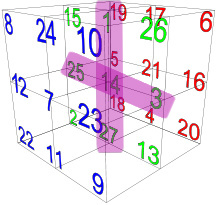Or Biblically, how was the Creator able to coordinate the Magic Cube so that the two crosshatches that are centered on David (14) each work out to 70, as in the 70 years of David’s life at the center of the time-line from Adam to Moshiach? For anyone that has not been following this series from its inception, you can find the entire work here.

### The Torah Matrix Explained

So how do we get the hyper complex Torah from the simple Magical Cube of 27 numbers, which all sum to 42. First, we convert the numbers to the 27 letters of the Hebrew Alef-bet using their ordinal values. Then using the 22-letter based Alef-bet, we determine all the possible Triplets derivable from them. These, by definition, are the number of permutations of 3 letters out of 22, given the possibility of repletion and a concern for specific order.  Simply, this works out to P’ (n,r) = nr = 223 = 10,648.  The next step is to arrange the 10,648 triplet permutations into a matrix of 248 columns by 42 rows, the Torah matrix, when the 16 blank rows between the Books are taken into account.  When the 22 letters are unwound and the Magic Cube of 42 spins out every possible Triplet, those 10,648 spun triplets, triangles and bars, form an exact 248 column by 42 row matrix, conforming to the exact dimensions of the Torah, and creating space, attraction points, and 3-d coordinates for every line in the Torah array.  Like every row and every column in the Cube of 27, the triplets line up in columns of 42 triplets each, completing exactly 248 columns.

Why 42? Well, that would be the secret of everything in the universe.

As stated earlier in this series 10,648 is the difference from the numbers of words, letters, verses, rows (10,400), and columns (248) in the Torah (401,273) and the number of words, letters, and verses in the Torah (390,625), an equation equivalent to exactly (248,000φ 58). And we also showed that the product of the digits in 390,625 divided by the sum of the digits in 390,625 = 1620/25 = 64.8, while the product of the digits in (390,625 and 401,273) divided by the sum of the digits in (390,625 and 401,273) = 272160/42 = 6480, or (10 x 648). While it is interesting that 1620, as the product of the digits in 390,625 is also the value of one of the Stars of David built into the Magic Cube of 42 and that is also the sum of the complete values of the 6 Matriarchs, there are deeper implications here.

There are 304,805 letters in the Torah and exactly 4,805 of them are the letter Pe(פ), leaving 300,000 of them for rest of the letters, which is equivalent to 1000 x 300, the value of the letter Shin(ש) X Binah (1000). On the rear face of the Cube we find the ordinal value (21) of Shin(ש) in the center and the ordinal value (17) of the letter Pe(פ) right above it.  There is more to it than that as 21 is the numerical value, and also the ordinal value, of the Name of G-d, Ehyeh (אהיה) associated with the hyperspace dimension of Binah.

The number of letters (304,805) in the Torah is 4,805 off from a perfect 300,000.  The number of words (79,975) in the Torah is off from a perfect 80,000 by a mere 25. The number of verses (5845) in the Torah is off by 155 from a perfect 6000. This makes the total a perfect 58. The total columns (248) and rows (42) are off by 10 from a perfect 300.

The total value that the Torah is purposely designed to be seemingly off from perfection is (4805 + 155 + 25 + 10), which equals 4995, the sum value of the 27 letters of the alef-bet and of the Magic Cube of 42.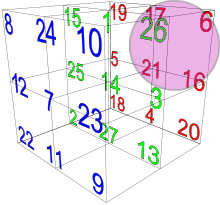The corresponding letters to that seeming perfection are Shin(ש) as 300, Pe(פ) as 80, and Vav(ו) as 6, as measured in thousands (386 x 1000). All 3 letters are found adjacent to one another on the “rear” face of the Magic Cube of 42.

### The 4 Aspects of the Tetragrammaton

There is more to it than that as 21 is the numerical value and also the ordinal value of the Name of G-d, Ehyeh (אהיה) associated with the hyperspace dimension of Binah.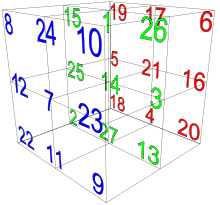We find that by natural design the 4 cubes adjacent (5 and 16; 17 and 4) to the 21 cube, also equal pairs of 21, making the crosshatch for this rear face, 63, as in the numerical value of the aspect of the Tetragrammaton associated with Binah: (יוד־הי־ואו־הי).  So does the diagonal (19-21-20) of the same face plus the kolel equal 63; these are also the coordinates of the splitting of the Red Sea beside the central Nun (נ) of ordinal value 14, in Exodus 14:19-21  The same can be said for the 4 corners (6, 20, 19, 18) that equal 63.  Those 4 corners break up into pairs as the front (26) and back (37) of the Name (יוד־הי־ואו־הי), an important Kabbalistic and spiritual distinction.  This “rear” face is indeed the face of Binah, which makes 2019 or 2018, 3330 years after the Spitting of the Red Sea, stand out all the more.

Like the rear face that includes the value 21, the top face includes the number 26, as in the value and ordinal value of the YHVH (יהוה).  We also have 3 different triangles on this top face that sum to 26: (19 – 1 –  6; 15 – 1 – 10; and 8 – 1 – 17), all pivoting off the central 1 (Alef), and covering all 4 directions. While 26 is the YHVH (יהוה), it is also the front (initials) of the aspect of the Tetragrammaton associated with Zeir Anpin: (יוד־הא־ואו־הא) of numerical value 45, just like the sum of the diagonals of this face. The back of this aspect is 19, as we discussed with Adam (45) and Eve (19), and thus we find that the ordinal value 19 is found as a corner in this “top” face. We also find that one of the diagonals (10 – 1 – 19) plus the adjacent ordinal value 15, also equals 45. What distinguishes this aspect of Zeir Anpin is that the letters in the Name are spelled out with Alefs(א) and that same diagonal when spelled out (יאק) equals 111, as in the numerical value of Alef(אלף). Thus this “top” face is indeed the face of Zeir Anpin.

Likewise, the “right” face also connects to Zeir Anpin in that the sum of its corners (10,9 and 6, 20) also equals 45, and they too break down into the pairs 26 and 19, the front and back of (יוד־הא־ואו־הא).

Remember, the directional order of the Cube’s faces is arbitrary. Any face can be designated right, left, or up, down, front or rear. Our particular usage of the terms is for reference to the orientation of our diagrams only.

### Simple Expansions

The complete numerical value of the central cube is 64 or 82 and when amplified through the 8 corners, giving us 88 or 16777216, which is 2563.  In other words, we get the value of the Ark of the Covenant cubed, which is also the inverse of the number of words, letters, and verses (58) in the Torah cubed. Remember, the Holy of Holies is a cube (203) and the Ark of the Covenant is in the center of the Holy of Holies, whether in the Mishkan or the Holy Temple.  Is it also a metaphor for the Seed of Creation?

Simple equations tell us that 122 = 144 and 28 = 256 and (12 + 8) = 20. Simple arithmetic then gives us (144 + 256) = 400 = 202 and (256144) = 112, as in the 112 Triplets. Continuing, (400 + 112) = 512 = 83.

Not so simple mathematics tell us this cube is an extraordinary projection of the 8 base dimensions and total 248 dimensions of the E8 Lattice.  The E8 root system consist of groups of 8 vectors (roots) that conform to specific parameters, with 112 of those groups being integers.  The 8 corners of the cube connect to these 8 vectors (roots) and the 112 Triplets connect to the 112 integer groups.  It is also not coincidental that (248 + 112) = 360, as in the degrees in a circle, an arbitrary number but one adapted by man and seemingly our universe.  Its usage dates back to Babylonia or even Mesopotamia.

### Negative Space

First, we must keep in mind that space, like time, is just a construct.  We were all taught that and space and even Einstein’s space-time is an actual fixed measurable commodity and that when the universe expands it is because matter and thus the galaxies are moving further away from the original big bang origin of the universe. The problem is that physicists no longer believe that.  It does not match up with their observations or measurements.  What happens in our physical universe, what they are observing, is that no matter where you are in the universe the stars and galaxies and all matter are moving away from you. That certainly dispels a big bang at a fixed original point singularity. It also makes gravity as we understand it unfathomable. It is almost as if the reality was that anti-gravity was the real norm. On the other hand, if you consider that space is not fixed and that the stars are not moving away from one another, but rather space itself is expanding, then the dynamic makes more sense. Welcome to the new reality.

Negative space, or inserting information in the apparent void between the letters, can tell us more than we know. The Kabbalists called this space white fire, as opposed to the letters, which were black fire. Given that we know the magic cube of 42 operates on the level of Binah and given all the connections in the 5 Essential elements to the number 1000, we can presume that each of the 27 cubes has a dimension of 1000, like the 1000 letters in the Shema, the 1000 average per row or column in the 8 x 8 Triplet matrix of 72, and the difference between the first verse of Bereshit and the 42-Letter Name.  That dimension would be 27 cubes of 10 x 10 x 10, or 103 each, arranged in a master cube of 33, quite apropos for the Tree-of-Life, or as described by the Kabbalists, 3 columns of 10 sefirot (dimensions) and their 10 sub-sefirot and 10 again sub-sefirot.

The structure of the master cube would thus be 30 x 30 x 30, for a volume of 27,000 overall. The value 30, as previously discussed connects to the letter Lamed(ל), which itself connects to the hyperspace dimensions of Binah, the Shofar, and the primal force or pressure mediation of the vortex. It is fitting, and also by design, that the complete value of the letter Lamed (ל) is 42.

Each 103 cube of 1000 would be comprised of a letter, black fire, and the “negative” space around it, white fire. This space is neither negative nor empty. It can be thought of for those that understand as the Ohr D’Chasidim (Light of Mercy) that surrounds and cloths the Ohr Chochma (Light of Wisdom).  Neither would exist without the other within this structure. The Ohr D’Chasidim (Light of Mercy) or surrounding light gives depth and structure and purpose to the Wisdom. That is the easier part of this concept. We must further understand that this Ohr D’Chasidim (Light of Mercy) or surrounding light is endless. It does not stop at the edge of a cube. The cube is a construct for our understanding, our comprehension. On the other hand, in Zeir Anpin and our world the Ohr D’Chasidim (Light of Mercy) is shaped by the letter (Light of Wisdom) inside it, as clothes are shaped by the body within. The Ohr Chochma (Light of Wisdom) reflected in each of the 27 letters is endless as well, yet what we are seeing, tapping into, are focal points, 27 focal points presented to us in a 3 x 3 x 3 array. The Ohr D’Chasidim (Light of Mercy) determines their arrangement.  These types of light should not be confused with Ohr Pnimi (Inner Light) and Ohr Makif (Encompassing or surrounding Light) as these pertain to the interaction of the Light with our consciousness.  At any rate, it is nomenclature and the concepts are what matters.

The letter Alef (א) in the top central block has the value of 1 and its negative space counterpart would thus be (10001) or 999. The letter Zadi sofit (ץ) in the bottom central block has the value of 900 and its negative space counterpart would thus be (1000900) or 100.  The numbers are assigned as references in our level of understanding; they do not reflect the endless capacity that each space really contains, making none greater or less than the next. Yet it is in reference to the Light in each particular letter that the surrounding light associated with that cube takes on a specific value itself. The sum total of those values of negative space for the entire Alef-bet, and thus the 27 cubes, is (27,0004995) = 22,005.

Very early on in this series, we discussed 5 chapters that were seemingly out of place chronologically within the Torah and within our overall analysis. We will repeat that section here because now we have the perspective to understand and appreciate why they were placed where they were and what they represent.

The 5 consecutive portions in the Book of Exodus that follow an incongruent time-line are Yitro, Mishpatim, Terumah, Tetzaveh, and Ki Thisa, and they stretch from Exodus 20 – 34; from paragraph 54 to 130; and from the 10 Commandments to the second set of Tablets.  The most astonishing thing quantitatively about those 5 parshot is not that they contain exactly 441 verses, as in the word emet, truth, but that they contain exactly 22,000 letters, as in (1000 x 22) letters, and as in the 22,005 negative space value for the 27 cubes of the Magic Cube of 42.

Those 5 portions that begin with the start of the 10 Commandments in the 70th chapter of the Torah at letter 107,007 and end with the 84th, just as Moses is about to carve the second set of Tablets, represents 7.2% of the entire Torah and spans 5788 words, 76 paragraphs and 15 chapters.  Then, while the total gematria of those 22,000 letters is 1733324, it is debatable whether the 173 reflects the 173 small gematria value of the 42-Letter Name in a similar way that the Top Quark having a mass of 173.07 GeV/c2 may or may not reflect it as well. Nonetheless, 1733324/26, the value of the Tetragrammaton (יהוה), is precisely 66666.308, an obvious design element of this special section that is aligned with the negative space in the Magic cube of 42, which has so many different elements that total 6660. Moreover, this span of 5 portions of the Torah’s 54 portions covers 18 columns of the Torah’s 248 total columns, and 756 total rows of the Torah’s 10,400 rows, just like the total ordinal value, 756, of the 6 faces of the cube.

In analyzing those 22,000 letters, we see that many of them reoccur a numerically significant number of times. It would be natural for one of them to, even two, but of the 22 letters, at least 7 of them do. For instance, the letter Bet (ב) reoccurs 1024 or 210 times, the same as the exact number of different word values in the entire Torah, which most significantly matches the 1024 edges of the 8-dimensional hypercube. Both the letter Gimmel (ג) and the letter Tet (ט) each reoccur 137 times, as in the numerical value of kabbalah, meaning “to receive” and the Fine Structure Constant (1/137), matching the sum up the cube roots of all 127 combinations of the Torah’s first verse, 1370.872. The letter Chet (ח) of numerical value 8 reoccurs 616 times amongst these 22,000, and that is the numerical value of H’Torah. It is also the exact number of times Moses’ exact name (משה) is mentioned in the Torah. It is also the value of Yitro(יתרו), his father-in-law, and the name of the first of the 5 portions, where the 10 Commandments are found. Then while the letter Pe(פ) reoccurs 358 times amongst those 22,000 letters, as in the numerical value of Mashiach(358), it breaks down to 316 normal Pes(פ) and 42 Pes sofit(ף). There are also 954 letter Ayin (ע) reoccurrences, as in digit #954 in Pi where we find the final of the three triplicate 5778, as previously discussed.

When we subtract the 22,000 letters in this special section from the 304,805 letters in the entire Torah, we get (30480522000) = 282805, aptly reminiscent of the square root of 8, or 2.82842…, and the square root of the exact number of words in the Torah: 282.8002828.

### The 112 Triplets Unwound

How does the Magic Cube of 42, consisting of the 27 Letters, interact with the 112 Triplets?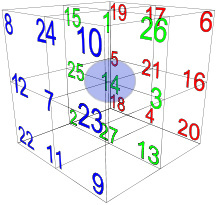Within Creation, there was first the Magic Cube of 42, consisting of the 27 Letters arranged in a very specific 33 array, according to their energy assignments.  Next the 336 letters of the 4 Essential Elements of Creation were spun out in 112 Triplets divided into 4 distinct matrices. It started with the central cube of the letter Nun (נ) of numerical value 14.  It divided or replicated into 14 letters Nun (נ) and they spread throughout the 112 Triplets.  They also match the total 14 sofit letters spread throughout the 112 Triplets.

One of the diagonals of the bottom face of the cube is (92718), and wedged between the number 9 cube and the number 27 cube is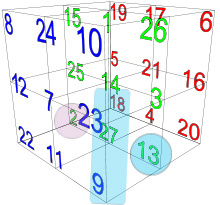the ordinal value 13 cube that stands for the letter Mem (מ). As it happens there are 9 Mem sofit (ם) spread throughout the 112 Triplets and 18 standard Mem (מ) for a total of 27 Mems (מ): (92718). Then opposite the 13 in the (13272) row is the ordinal value 2 for the letter Bet (ב), as in the letter pair (מב) or 42. Opposite the letter Bet (ב) vertically in the (22515) column we find the Samech (ס) of ordinal value 15 and there are 2 letter Samech (ס) in all of the 112 Triplets.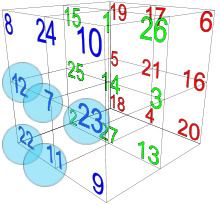In the “front” face of the cube , we see that there are 11 Tavs(ת) of ordinal value 22 spread throughout the 112 Triplets, and the ordinal value of the number of the letter to the right of the letter Tav(ת) is 11, which in turn is the ordinal value for Caf (כ), and right above Caf (כ) is the value 7 for the 7 Cafs (כ) spread throughout the 112 Triplets. Next to the letter Tav(ת) on the other side is the letter Lamed (ל) of ordinal value 12, and right across from it on the same “front” face is number 23, matching the 23 Lameds (ל) spread throughout the 112 Triplets. As we will understand shortly, the number 23 in this interlocking equation is the designed result of (11 + 12).

If instead of crossing the face, we diagonally cross the cube with the sequential (121416) diagonal we find that there are 12 letters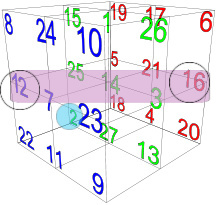Ayin (ע) of ordinal value 16 spread throughout the 112 Triplets.  Like the letter Ayin (ע), the letter Bet (ב) is also found 12 times throughout the 112 Triplets and the number 12 cube is diagonally attached to it on the “left” face.

Back to the “front” face, where we find that across the (978) diagonal there are 9 Chets (ח) of ordinal value 8 spread amongst the 112 Triplets.  While there are 7 Cafs (כ) in the 112 Triplets, when we include the 1 sofit one, there are a total of 8 Cafs (כ), the next number up in the (879) diagonal.  Breaking the pattern slightly concerning the same (879) diagonal, there are a total of 8, four (4) each, letters Tzion (ז) and Tet (ט) of ordinal values 7 and 9 respectively throughout the 112 Triplets. Speaking of 4, there are 11 letters Dalet (ד) of ordinal value 4, and directly across the cube from the letter Dalet (ד) is number 11. Moreover, next to the letter Dalet (ד) is the letter Shin (ש), also found 11 times in the 112 Triplets.

There are four (4) Essential Elements of Creation that consist of 112 Triplets.  Notice which letters reoccur 11 and 12 times in those 112 Triplets, or that have ordinal values of 11 or 12, and how they are all interconnected.  It appears to be more of a hidden message to us rather than a necessary design feature, though that too may be true on a higher level or dimension beyond our comprehension. Those 6 letters (שבתכלד) can obviously permute to many different words and values, but in total they equal 756. Yes, the same 756 as in the total ordinal value, 756, of the 6 faces of the cube, and as in the base of the Pyramid, the spelled-out letter Nun(נון), the value of (18 x 42), etc. Their total ordinal value is 72.

When the letter Ayin (ע) is added as a 7th letter (שבכלדעת) the permutations go up exponentially, yet their total ordinal value is 88 and their total occurrences within the 112 Triplets is 88.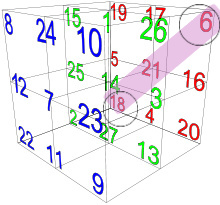Then going back to the previous pattern, but on the opposite face, is diagonal (18216), where we find that the letter Zadi (צ) of ordinal value 18 is found 6 times throughout the 112 Triplets and thus linked to the ordinal value 6 at the opposite end of the diagonal, a pattern seen numerous times already.

Two other letters that correlate between the “rear” and “front” faces are the 7 letters Koof (ק) of ordinal value 19 in the rear face corresponding to number 7 in the front face.  Then the 15 letters Resh (ר) of ordinal value 20 corresponds to the combined numbers 7 and 8 of the front face.

Of more complex correlation are the 41 letters Hei (ה) of ordinal value 5 in the rear face, which corresponds with the Triplet (82211) in the front face; and then the 37 letters Vav (ו) of ordinal value 6 in the rear face, which corresponds with several Triplet combinations in the front face.  There is also the 53 letters Yud (י) of ordinal value 10 in the front face that correspond with the Triplet (191816) in the rear face. These 3 letters of the Tetragrammaton (יהוה) occur a total of (37 + 53 + 41) = 131 times and when combined with the complete numerical value of the 3 letters (יהו), which is 42, equals 173, as in the small gematria of the 42-Letter Name.

The only 3 letters that do not have direct correlations are the 22 Alefs (א), the 4 Gimmels (ג) and the 3 Pes (פ) and they (אגפ) have a total value of 84. On the other hand, the 22 Alefs (א) breakdown to 7 Alefs (א) in Bereshit, 14 Alefs (א) in the 72 Triplets and 1 in the 42-Letter Name line up with the (7141) contiguous right-angle triangle through the center of the cube. Also, the 1 Gimmel (ג) in the 72 Triplets and the 3 Gimmels (ג) in the 42-Letter Name line up with the 13 combination also through the center of the cube.

The “front” face of the cube, as we have said previously, is the face of 7’s with 7 in the central position, 72 as the 4 corners and 77 as the sum of the 4 axial points. By divine design, the sum of the 112 Triplet occurrences of the 4 corners is also 77, and because the crosshatch letter recurrences are 44, the total recurrences for the “front” face letters is 112.

### A Rubik’s Cube

The 112 Triplets were created for energetic purposes, for their relationships between pressure mediations and the primordial forces of the upper dimensions.  They were created, as we have previously seen, with mathematical precision, reflecting the primordial constants as we know them.  They were created so our world could exist.  Any patterns linking them to the 5th and really the first Essential Element of Creation, the Magic Cube of 42 or Bird’s Nest reflects their ties to the infinite, the endless, more than a way for us to unravel them.  They are to be understood as Triplets.

We live in the world of Tikune, spelled out for us as the diagonal of the left face (תקן) 22 – 25 – 19, a world of correction.  The Cube exists in a world of perfection. The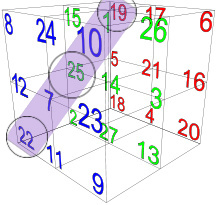112 Triplets help us to bridge those worlds.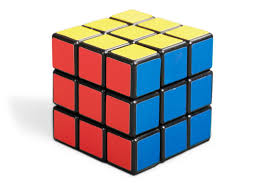The 11 Triplets of Bereshit is a real Rubik’s cube with each pair of letters in the Torah’s first word (בר־אש־ית) found in a different plane of the cube. Nevertheless, all 11 Triplets individually can be found on a single face at a time with the right axial rotations.

The 72 Triplets start out on the “rear” face and can all be constructed using similar Rubik’s cube machinations.

All 15 Triplets of the Shema with one turn of the bottom plane to place the Mem (מ) on the rear face can be wholly constructed on that same “rear” face.

And the 14 Triplets of the 42 Letter Name start out (אבג) or (123) in the central vertical plane like a trifold around the most central 14. The 14 Triplets can all also be constructed around a single face, plane, or tight triangle with the central Nun(נ), except for one, the Triplet associated with satan (שטן). This is the only one within the 112 Triplets that cannot be constructed in this natural fashion. Do not for a second think this is a coincident.

Think of the Magical Cube of 42 as the mother ship and the Triplets as satellites that can operate as a hive (collective), in specific groups, or independently. For anyone that has not been following this series from its inception, you can find the entire work here.